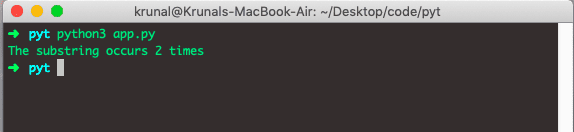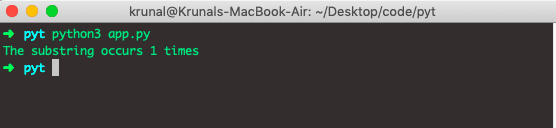# Python String count() Method

Python string count() is a built-in function that “returns the number of occurrences of the substring in the given string”. The method searches the substring in the given string and returns how many times it is present. It takes optional parameters to start and end to specify the starting and ending positions in the string, respectively.

### Syntax

``string.count(substring, start, end)``

### Parameters

The count() function has one compulsory and two optional parameters.

The substring is a required parameter, a string whose count is to be found.

The start parameter is Optional, the starting index within the string where the search starts.

The end parameter is Optional, the ending index within the string where the search ends.

### Return Value

Python String count() method returns an integer that denotes the number of times a substring occurs in a given string.

### Example 1

``````friend = 'What did you bring Mr. Bing'
substring = 'ing'
count = friend.count(substring)

print('The substring occurs {} times'.format(count))``````

Here, we have used the ing as a substring and checked how often it is repeated inside the String. Also, we used the String.format() to format the string.

Output### Example 2

Let’s take an example where we pass a start and end parameter.

``````friend = 'What did you bring Mr. Bing'
substring = 'ing'
count = friend.count(substring, 11, 18)

print('The substring occurs {} times'.format(count))``````

OutputIt will count the number of substrings between the 11th and 18th index characters. In our example, ing occurs between those characters only once, so we see the one time.

That’s it.

This site uses Akismet to reduce spam. Learn how your comment data is processed.